# Factoring PolynomialsPage 8

#### WATCH ALL SLIDES

a, b and c are real numbers and a  0.

This is referred to as standard form.

Zero Factor Theorem

If a and b are real numbers and ab = 0, then a = 0 or b = 0.

This theorem is very useful in solving quadratic equations.

Slide 51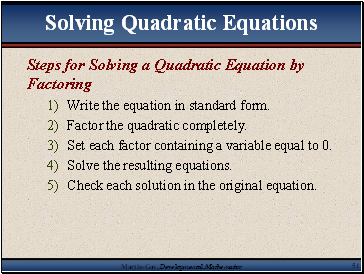Steps for Solving a Quadratic Equation by Factoring

Write the equation in standard form.

Set each factor containing a variable equal to 0.

Solve the resulting equations.

Check each solution in the original equation.

Slide 52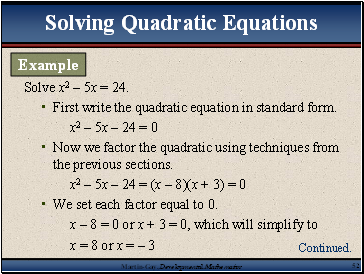Solve x2 – 5x = 24.

First write the quadratic equation in standard form.

x2 – 5x – 24 = 0

Now we factor the quadratic using techniques from the previous sections.

x2 – 5x – 24 = (x – 8)(x + 3) = 0

We set each factor equal to 0.

x – 8 = 0 or x + 3 = 0, which will simplify to

x = 8 or x = – 3

Example

Continued.

Slide 53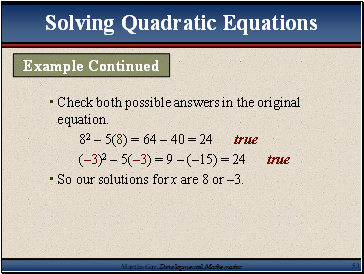Check both possible answers in the original equation.

82 – 5(8) = 64 – 40 = 24 true

(–3)2 – 5(–3) = 9 – (–15) = 24 true

So our solutions for x are 8 or –3.

Example Continued

Slide 54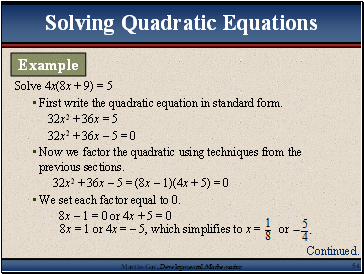Solve 4x(8x + 9) = 5

First write the quadratic equation in standard form.

32x2 + 36x = 5

32x2 + 36x – 5 = 0

Now we factor the quadratic using techniques from the previous sections.

32x2 + 36x – 5 = (8x – 1)(4x + 5) = 0

We set each factor equal to 0.

8x – 1 = 0 or 4x + 5 = 0

Example

Continued.

Slide 55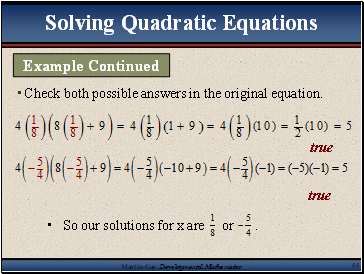Check both possible answers in the original equation.

Example Continued

Slide 56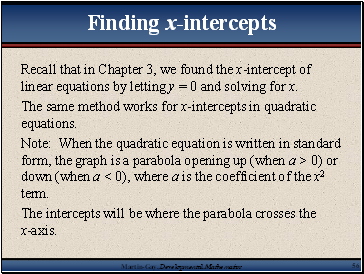## Finding x-intercepts

Recall that in Chapter 3, we found the x-intercept of linear equations by letting y = 0 and solving for x.

The same method works for x-intercepts in quadratic equations.

Note: When the quadratic equation is written in standard form, the graph is a parabola opening up (when a > 0) or down (when a < 0), where a is the coefficient of the x2 term.

The intercepts will be where the parabola crosses the x-axis.

Slide 57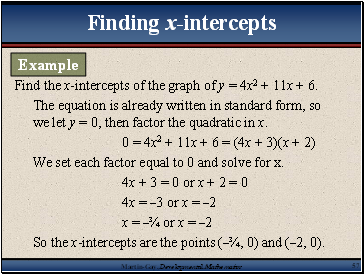Find the x-intercepts of the graph of y = 4x2 + 11x + 6.

The equation is already written in standard form, so we let y = 0, then factor the quadratic in x.

0 = 4x2 + 11x + 6 = (4x + 3)(x + 2)

Go to page:
1  2  3  4  5  6  7  8  9  10